Publicité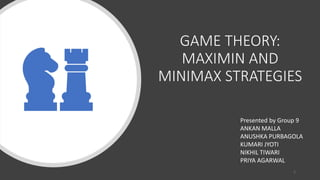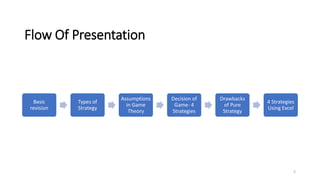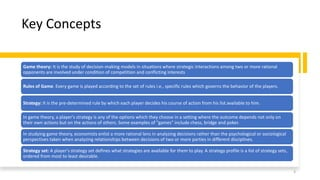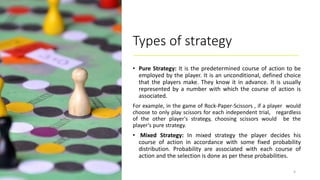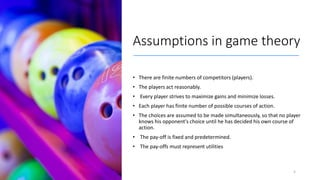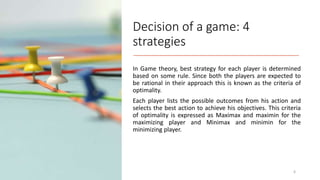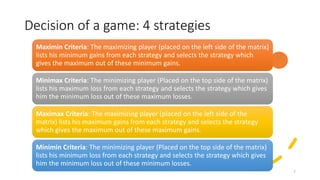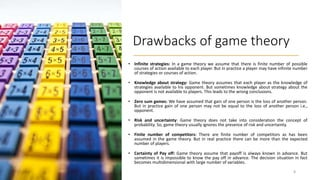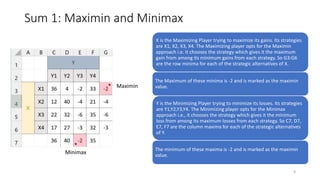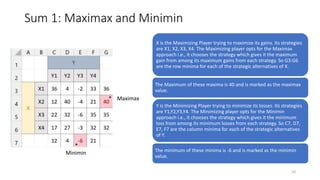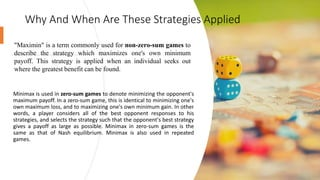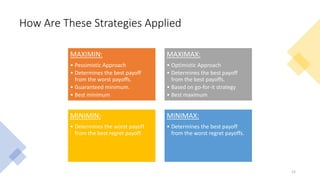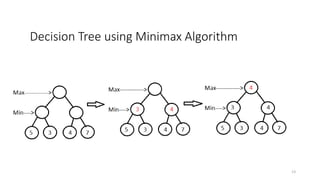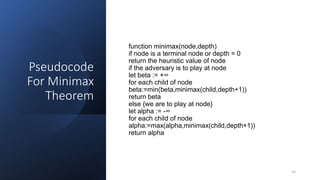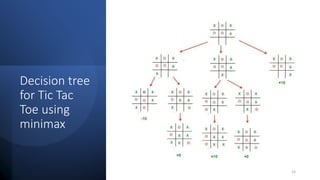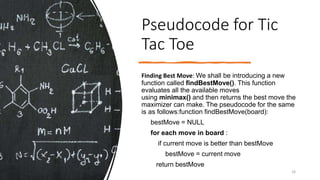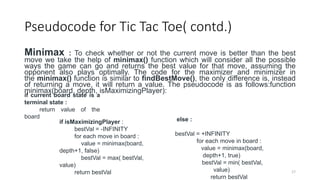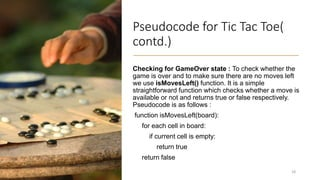1 sur 20
Publicité

### OR PPT 280322 maximin final - nikhil tiwari.pptx

1. GAME THEORY: MAXIMIN AND MINIMAX STRATEGIES Presented by Group 9 ANKAN MALLA ANUSHKA PURBAGOLA KUMARI JYOTI NIKHIL TIWARI PRIYA AGARWAL 1
2. Flow Of Presentation Basic revision Types of Strategy Assumptions in Game Theory Decision of Game- 4 Strategies Drawbacks of Pure Strategy 4 Strategies Using Excel 2
3. Game theory: It is the study of decision-making models in situations where strategic interactions among two or more rational opponents are involved under condition of competition and conflicting interests Rules of Game. Every game is played according to the set of rules i.e., specific rules which governs the behavior of the players. Strategy: It is the pre-determined rule by which each player decides his course of action from his list available to him. In game theory, a player's strategy is any of the options which they choose in a setting where the outcome depends not only on their own actions but on the actions of others. Some examples of "games" include chess, bridge and poker. In studying game theory, economists enlist a more rational lens in analyzing decisions rather than the psychological or sociological perspectives taken when analyzing relationships between decisions of two or more parties in different disciplines. Strategy set: A player's strategy set defines what strategies are available for them to play. A strategy profile is a list of strategy sets, ordered from most to least desirable. Key Concepts 3
4. Types of strategy • Pure Strategy: It is the predetermined course of action to be employed by the player. It is an unconditional, defined choice that the players make. They know it in advance. It is usually represented by a number with which the course of action is associated. For example, in the game of Rock-Paper-Scissors , if a player would choose to only play scissors for each independent trial, regardless of the other player's strategy, choosing scissors would be the player's pure strategy. • Mixed Strategy: In mixed strategy the player decides his course of action in accordance with some fixed probability distribution. Probability are associated with each course of action and the selection is done as per these probabilities. 4
5. Assumptions in game theory • There are finite numbers of competitors (players). • The players act reasonably. • Every player strives to maximize gains and minimize losses. • Each player has finite number of possible courses of action. • The choices are assumed to be made simultaneously, so that no player knows his opponent's choice until he has decided his own course of action. • The pay-off is fixed and predetermined. • The pay-offs must represent utilities 5
6. Decision of a game: 4 strategies In Game theory, best strategy for each player is determined based on some rule. Since both the players are expected to be rational in their approach this is known as the criteria of optimality. Each player lists the possible outcomes from his action and selects the best action to achieve his objectives. This criteria of optimality is expressed as Maximax and maximin for the maximizing player and Minimax and minimin for the minimizing player. 6
7. Decision of a game: 4 strategies Maximin Criteria: The maximizing player (placed on the left side of the matrix) lists his minimum gains from each strategy and selects the strategy which gives the maximum out of these minimum gains. Minimax Criteria: The minimizing player (Placed on the top side of the matrix) lists his maximum loss from each strategy and selects the strategy which gives him the minimum loss out of these maximum losses. Maximax Criteria: The maximizing player (placed on the left side of the matrix) lists his maximum gains from each strategy and selects the strategy which gives the maximum out of these maximum gains. Minimin Criteria: The minimizing player (Placed on the top side of the matrix) lists his minimum loss from each strategy and selects the strategy which gives him the minimum loss out of these minimum losses. 7
8. Drawbacks of game theory • Infinite strategies: In a game theory we assume that there is finite number of possible courses of action available to each player. But in practice a player may have infinite number of strategies or courses of action. • Knowledge about strategy: Game theory assumes that each player as the knowledge of strategies available to his opponent. But sometimes knowledge about strategy about the opponent is not available to players. This leads to the wrong conclusions. • Zero sum games: We have assumed that gain of one person is the loss of another person. But in practice gain of one person may not be equal to the loss of another person i.e., opponent. • Risk and uncertainty: Game theory does not take into consideration the concept of probability. So, game theory usually ignores the presence of risk and uncertainty. • Finite number of competitors: There are finite number of competitors as has been assumed in the game theory. But in real practice there can be more than the expected number of players. • Certainty of Pay off: Game theory assume that payoff is always known in advance. But sometimes it is impossible to know the pay off in advance. The decision situation in fact becomes multidimensional with large number of variables. 8
9. Sum 1: Maximin and Minimax * Maximin * Minimax X is the Maximizing Player trying to maximize its gains. Its strategies are X1, X2, X3, X4. The Maximizing player opts for the Maximin approach i.e. it chooses the strategy which gives it the maximum gain from among its minimum gains from each strategy. So G3:G6 are the row minima for each of the strategic alternatives of X. The Maximum of these minima is -2 and is marked as the maximin value. Y is the Minimizing Player trying to minimize its losses. Its strategies are Y1,Y2,Y3,Y4. The Minimizing player opts for the Minimax approach i.e., it chooses the strategy which gives it the minimum loss from among its maximum losses from each strategy. So C7, D7, E7, F7 are the column maxima for each of the strategic alternatives of Y. The minimum of these maxima is -2 and is marked as the maximin value. 9
10. Sum 1: Maximax and Minimin * Maximax * Minimin X is the Maximizing Player trying to maximize its gains. Its strategies are X1, X2, X3, X4. The Maximizing player opts for the Maximax approach i.e., it chooses the strategy which gives it the maximum gain from among its maximum gains from each strategy. So G3:G6 are the row minima for each of the strategic alternatives of X. The Maximum of these maxima is 40 and is marked as the maximax value. Y is the Minimizing Player trying to minimize its losses. Its strategies are Y1,Y2,Y3,Y4. The Minimizing player opts for the Minimin approach i.e., it chooses the strategy which gives it the minimum loss from among its minimum losses from each strategy. So C7, D7, E7, F7 are the column minima for each of the strategic alternatives of Y. The minimum of these minima is -6 and is marked as the minimin value. 10
11. Minimax is used in zero-sum games to denote minimizing the opponent's maximum payoff. In a zero-sum game, this is identical to minimizing one's own maximum loss, and to maximizing one's own minimum gain. In other words, a player considers all of the best opponent responses to his strategies, and selects the strategy such that the opponent's best strategy gives a payoff as large as possible. Minimax in zero-sum games is the same as that of Nash equilibrium. Minimax is also used in repeated games. Why And When Are These Strategies Applied "Maximin" is a term commonly used for non-zero-sum games to describe the strategy which maximizes one's own minimum payoff. This strategy is applied when an individual seeks out where the greatest benefit can be found.
12. How Are These Strategies Applied MAXIMIN: • Pessimistic Approach • Determines the best payoff from the worst payoffs. • Guaranteed minimum. • Best minimum MAXIMAX: • Optimistic Approach • Determines the best payoff from the best payoffs. • Based on go-for-it strategy • Best maximum MINIMIN: • Determines the worst payoff from the best regret payoff. MINIMAX: • Determines the best payoff from the worst regret payoffs. 12
13. Decision Tree using Minimax Algorithm 13
14. Pseudocode For Minimax Theorem function minimax(node,depth) if node is a terminal node or depth = 0 return the heuristic value of node if the adversary is to play at node let beta := +∞ for each child of node beta:=min(beta,minimax(child,depth+1)) return beta else {we are to play at node} let alpha := -∞ for each child of node alpha:=max(alpha,minimax(child,depth+1)) return alpha 14
15. Decision tree for Tic Tac Toe using minimax 15
16. Pseudocode for Tic Tac Toe Finding Best Move: We shall be introducing a new function called findBestMove(). This function evaluates all the available moves using minimax() and then returns the best move the maximizer can make. The pseudocode for the same is as follows:function findBestMove(board): bestMove = NULL for each move in board : if current move is better than bestMove bestMove = current move return bestMove 16
17. Pseudocode for Tic Tac Toe( contd.) Minimax : To check whether or not the current move is better than the best move we take the help of minimax() function which will consider all the possible ways the game can go and returns the best value for that move, assuming the opponent also plays optimally. The code for the maximizer and minimizer in the minimax() function is similar to findBestMove(), the only difference is, instead of returning a move, it will return a value. The pseudocode is as follows:function minimax(board, depth, isMaximizingPlayer): else : bestVal = +INFINITY for each move in board : value = minimax(board, depth+1, true) bestVal = min( bestVal, value) return bestVal if isMaximizingPlayer : bestVal = -INFINITY for each move in board : value = minimax(board, depth+1, false) bestVal = max( bestVal, value) return bestVal if current board state is a terminal state : return value of the board 17
18. Pseudocode for Tic Tac Toe( contd.) Checking for GameOver state : To check whether the game is over and to make sure there are no moves left we use isMovesLeft() function. It is a simple straightforward function which checks whether a move is available or not and returns true or false respectively. Pseudocode is as follows : function isMovesLeft(board): for each cell in board: if current cell is empty: return true return false 18
19. References • https://www.geeksforgeeks.org/minimax- algorithm-in-game-theory-set-3-tic-tac-toe- ai-finding-optimal-move/ • https://en.m.wikipedia.org/wiki/Minimax • https://www.researchgate.net/figure/Flowch art-for-minimax-algorithm_fig1_331552609 19
20. Thank you 20
Publicité